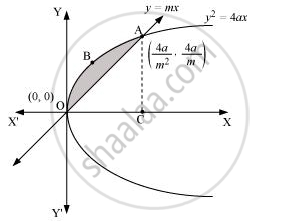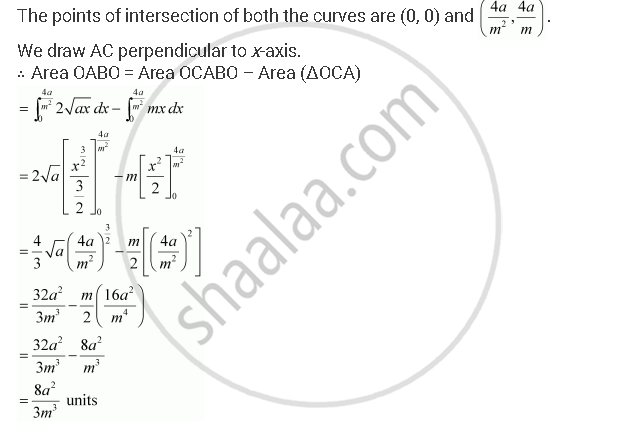Share

# Find the Area Enclosed Between the Parabola Y2 = 4ax and the Line Y = Mx - Mathematics

#### Question

Find the area enclosed between the parabola y2 = 4ax and the line y mx

#### Solution

The area enclosed between the parabola, y2 = 4ax, and the line, y mx, is represented by the shaded area OABO asIs there an error in this question or solution?